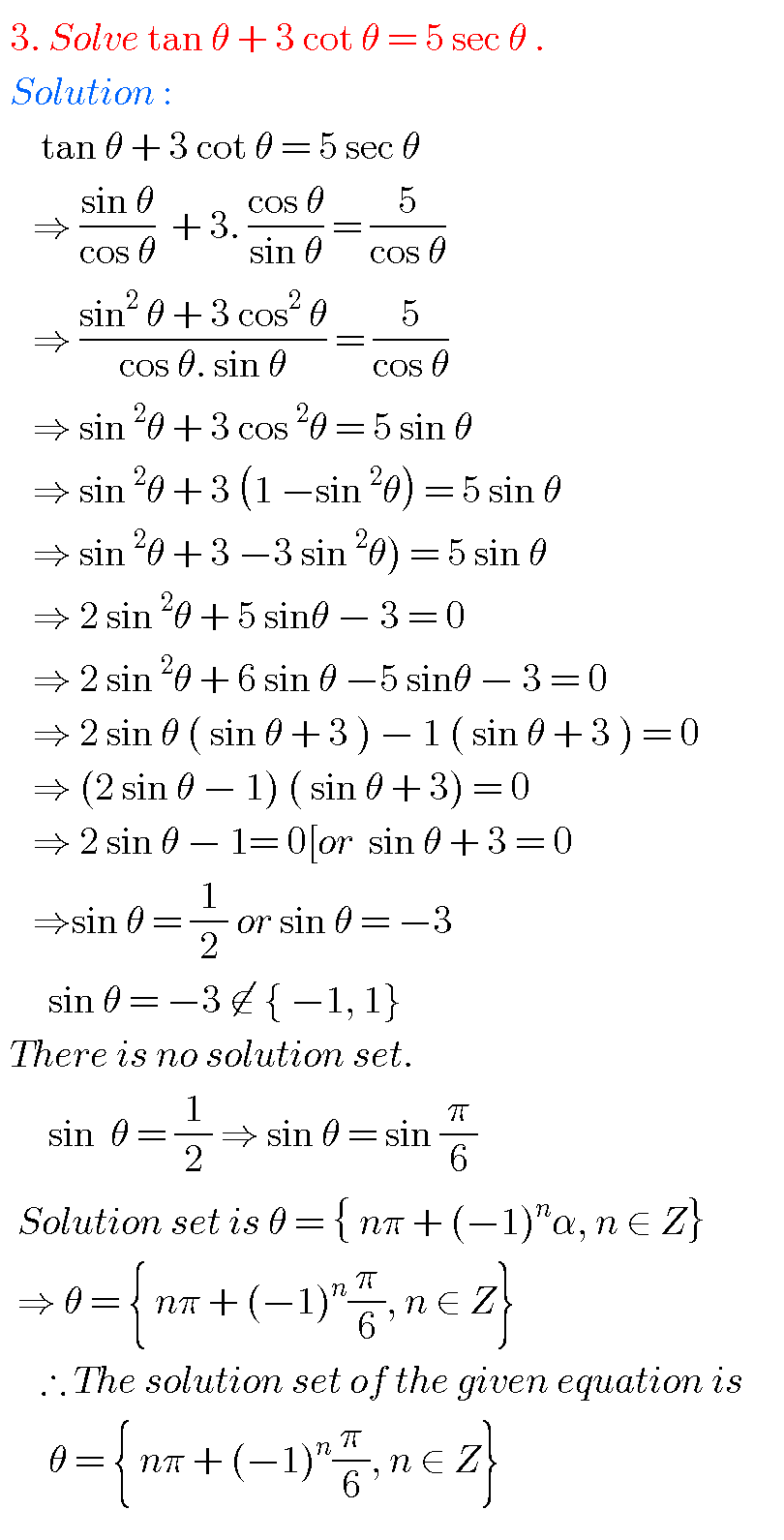# TRIGONOMETRIC EQUATIONS, INTERMEDIATE FIRST YEAR 1 A CHAPTER 7 PROBLEMS WITH SOLUTIONS

Mathematics intermediate 1A  solutions for some problems.

These solutions are very simple to understand.

Study the textbook lesson Trigonometric Equations very well.

Observe the example problems and solutions given in the textbook.

Text book exercise solutions links are also given.

Trigonometric Equations

Exercise 7(a)

You can also see

Inter Maths 1A textbook solutions

Inter Maths 1B textbook solutions

Inter Maths IIA textbook solutions

Inter Maths IIB textbook solutions

Nios solutions for maths 311 book 1 Sets 1.1

M

### Trigonometric equations problems with solutionsNote : Observe the solutions of trigonometric equations and try them in your own methods.

Some more solutionsYou can see the solutions for Junior Inter maths 1A

3. Matrices

You can also see

Ncert solutions for maths class 6 th some chapters

Ncert solutions for maths class 7 th some chapters

Ncert solutions for maths class 8 some chapters

Maths solutions class 10 real numbers

### 1 thought on “Trigonometric Equations,Intermediate Mathematics first year 1 A chapter 7 problems with solutions,Junior inter Trigonometry”

1.I really like this and thank you 😊😊😊😊😊😊😊😊😊